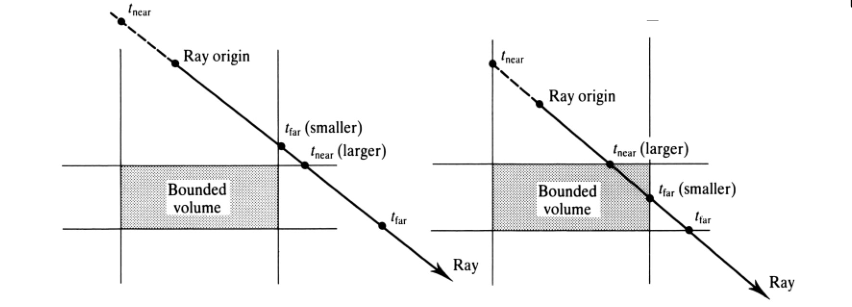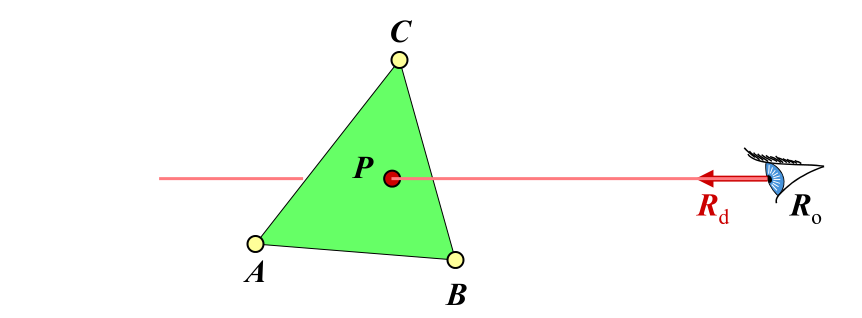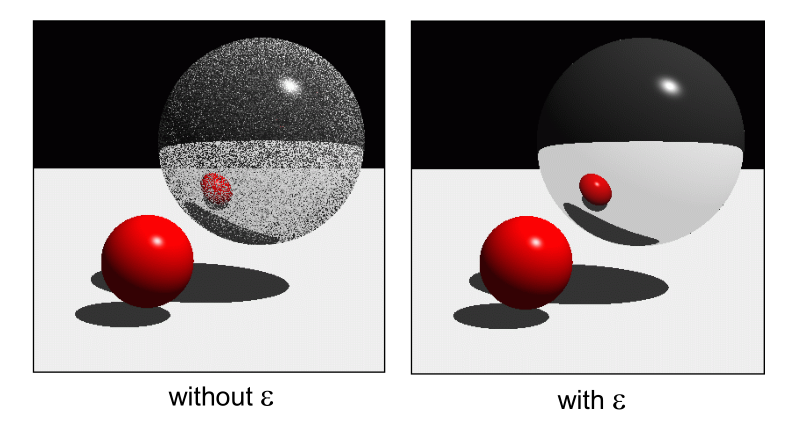# Various surfaces

## Ray-Plane Intersection

Plane is often represented in implicit form :

Equivalent:

where $N = [A B C]^T$ and $P = [x y z]^T$

To find ray-plane intersection, substitute ray equation $P(t)$ into plane equation:

1. We get $N \cdot P + D = 0$.
2. Sovle for $t$ to get $t_0$.
3. if $t_0$ is infinity, no intersection (ray is parallel to plane).
4. Intersection point is $P(t_0)$.
5. Verify that intersection is not behind ray origin.

The normal at the intersection is $N$ (or $-N$)

## Ray-Sphere Intersection

Sphere (centered at origin) is often represented in implicit form:

Equivalent:

To find ray-plane intersection, substitute ray equation P(t) into plane equation:

We get $P \cdot P - r^2 = 0$:

$R_o$ is ray origin, $R_d$ is ray direction.

It is a quadratic equation in the form $at^2 + bt + c = 0$

• $a = R_o \cdot R_o = 1$ (Since $|R_d| = 1$)
• $b = 2R_d \cdot R_o$
• $c = R_o \cdot R_o - r^2$

Discriminant: $d = b^2 + 4ac$

Solution: $t_\pm = \frac{-b\pm\sqrt{b^2 + 4ac}}{2a}$

Choose $t_0$ as the closest positive $t$ value ($t_+$ + or $t_-$)

The normal at the intersection point is $P(t_0)/|P(t_0)|$

Very easy to compute, that is why most ray tracing images have spheres.

## Ray-Box Intersection

To find ray-box intersection:

• For each pair of parallel plane, find the distance to the first plane ($t_{near}$) and to the second plane ($t_{far}$).
• Keep the largest $t_{near}$ so far, and smallest $t_{far}$ so far.
• If largest $t_{near}$ > smallest $t_{far}$ , no intersection.
• Otherwise, the intersection is at P(largest $t_{near}$ )## Ray-Triangle Intersection

Finding intersection between a ray and a general polygon is difficult.

• Compute ray-plane intersection
• Determine whether intersection is within polygon
• Tedious for non-convex polygon
• Interpolation of attributes at the vertices are not well-
defined

Much easier to find ray-triangle intersection

• Can use the barycentric coordinates method.
• Interpolation of attributes at the vertices are well-defined using the barycentric coordinates.

### Barycentric Coordinates

The barycentric coordinates of a point P on a triangle $ABC$ is ($\alpha$, $\beta$, $\gamma$) such that:

where

and

We can rewrite it as:To find ray-triangle intersection, we let:

Solve for $t$, $\beta$ and $\gamma$

Intersection if $\beta + \gamma < 1$ and $\beta,\gamma > 0$ and $t > 0$

Expand &R_o + tR_d = A + \beta(B-A) + \gamma(C-A)&

we have 3 equations and 3 unknowns here.

Regroup and write in matrix form

Use Cramer’s Rule to solve for $t$, $\beta$ and $\gamma$

• Efficient
• No need to store plane equation
• Barycentric coordinates are useful for linear interpolation of normal vectors, texture coordinates, and other attributes at the vertices

For example, the interpolated normal at $P$ is:

and all vector should do a normalization.

## The “Epsilon” Problem

Should not accept intersection for very small positive $t$

• May falsely intersect the surface at the ray origin
• Method 1: Use an epsilon value $\varepsilon$ > 0, and accept an intersection only if its $t > \varepsilon$.
• Method 2: When a new ray is spawned, advanced the ray origin by an epsilon distance $\varepsilon$ in the ray directionLearn OpenGL

Share